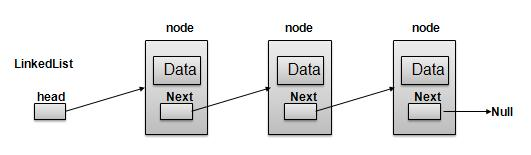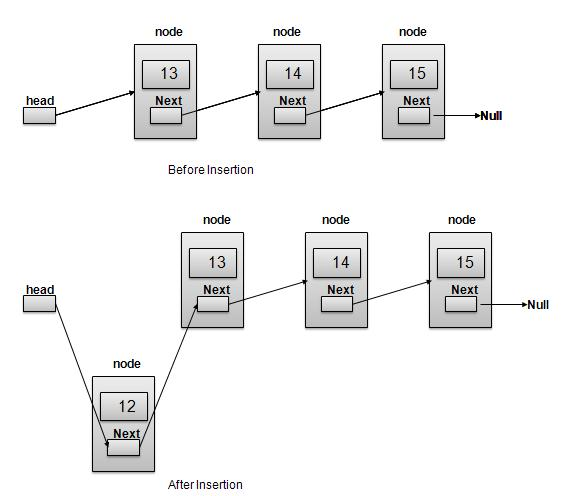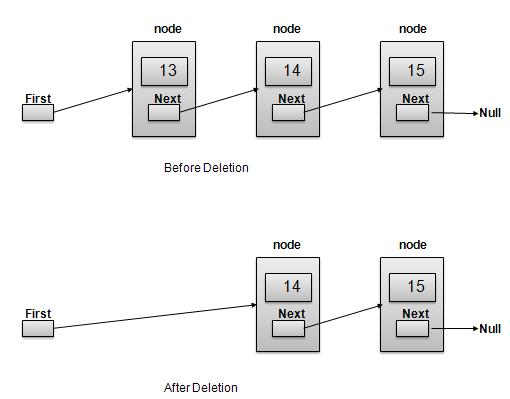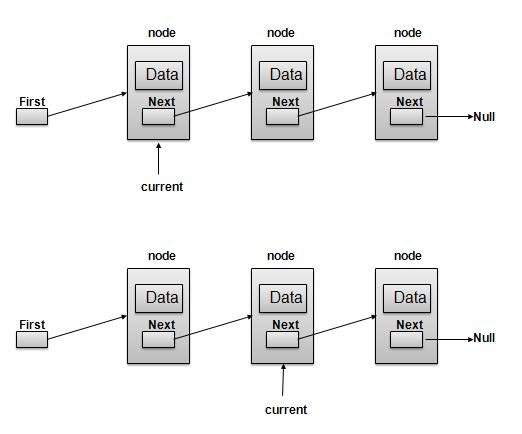# DSA using C - Linked List

#### Complete Python Prime Pack

9 Courses     2 eBooks

#### Artificial Intelligence & Machine Learning Prime Pack

6 Courses     1 eBooks

#### Java Prime Pack

9 Courses     2 eBooks

## Overview

Linked List is a sequence of links which contains items. Each link contains a connection to another link. Linked list the second most used data structure after array. Following are important terms to understand the concepts of Linked List.

• Link − Each Link of a linked list can store a data called an element.

• Next − Each Link of a linked list contain a link to next link called Next.

• LinkedList − A LinkedList contains the connection link to the first Link called First.

## Linked List RepresentationAs per above shown illustration, following are the important points to be considered.

• LinkedList contains an link element called first.

• Each Link carries a data field(s) and a Link Field called next.

• Last Link carries a Link as null to mark the end of the list.

## Types of Linked List

Following are the various flavours of linked list.

• Simple Linked List − Item Navigation is forward only.

• Doubly Linked List − Items can be navigated forward and backward way.

• Circular Linked List − Last item contains link of the first element as next and and first element has link to last element as prev.

## Basic Operations

Following are the basic operations supported by a list.

• Insertion − add an element at the beginning of the list.

• Deletion − delete an element at the beginning of the list.

• Display − displaying complete list.

• Search − search an element using given key.

• Delete − delete an element using given key.

## Insertion Operation

Insertion is a three step process −

• Create a new Link with provided data.

• Point New Link to old First Link.

• Point First Link to this New Link.```//insert link at the first location
void insertFirst(int key, int data){
struct node *link = (struct node*) malloc(sizeof(struct node));

//point it to old first node

//point first to new first node
}
```

## Deletion Operation

Deletion is a two step process −

• Get the Link pointed by First Link as Temp Link.```//delete first item
struct node* deleteFirst(){
//save reference to first link

//mark next to first link as first

//return the deleted link
}
```

Navigation is a recursive step process and is basis of many operations like search, delete etc. −

• Get the Link pointed by First Link as Current Link.

• Check if Current Link is not null and display it.

• Point Current Link to Next Link of Current Link and move to above step.Note −

```//display the list
void printList(){
struct node *ptr = head;
printf("\n[ ");

//start from the beginning
while(ptr != NULL){
printf("(%d,%d) ",ptr->key,ptr->data);
ptr = ptr->next;
}
printf(" ]");
}
```

Following are the advanced operations specified for a list.

• Sort − sorting a list based on a particular order.

• Reverse − reversing a linked list.

## Sort Operation

We've used bubble sort to sort a list.

```void sort(){
int i, j, k, tempKey, tempData ;
struct node *current;
struct node *next;
int size = length();
k = size ;
for ( i = 0 ; i < size - 1 ; i++, k-- ) {
current = head ;
next = head->next ;
for ( j = 1 ; j < k ; j++ ) {
if ( current->data > next->data ) {
tempData = current->data ;
current->data = next->data;
next->data = tempData ;

tempKey = current->key;
current->key = next->key;
next->key = tempKey;
}
current = current->next;
next = next->next;
}
}
}
```

## Reverse Operation

Following code demonstrate reversing a single linked list.

```void reverse(struct node** head_ref) {
struct node* prev   = NULL;
struct node* current = *head_ref;
struct node* next;
while (current != NULL) {
next  = current->next;
current->next = prev;
prev = current;
current = next;
}
}
```

## Example

```#include <stdio.h>
#include <string.h>
#include <stdlib.h>
#include <stdbool.h>

struct node {
int data;
int key;
struct node *next;
};
struct node *head = NULL;
struct node *current = NULL;

//display the list
void printList(){
struct node *ptr = head;
printf("\n[ ");

//start from the beginning
while(ptr != NULL){
printf("(%d,%d) ",ptr->key,ptr->data);
ptr = ptr->next;
}
printf(" ]");
}
//insert link at the first location
void insertFirst(int key, int data){
struct node *link = (struct node*) malloc(sizeof(struct node));

//point it to old first node

//point first to new first node
}
//delete first item
struct node* deleteFirst(){
//save reference to first link

//mark next to first link as first

//return the deleted link
}
//is list empty
bool isEmpty(){
return head == NULL;
}
int length(){
int length = 0;
struct node *current;
for(current = head; current!=NULL;
current = current->next){
length++;
}
return length;
}
//find a link with given key
struct node* find(int key){
//start from the first link
struct node* current = head;

//if list is empty
return NULL;
}
//navigate through list
while(current->key != key){
//if it is last node
if(current->next == NULL){
return NULL;
} else {
//go to next link
current = current->next;
}
}
//if data found, return the current Link
return current;
}
//delete a link with given key
struct node* delete(int key){
//start from the first link
struct node* current = head;
struct node* previous = NULL;

//if list is empty
return NULL;
}
//navigate through list
while(current->key != key){
//if it is last node
if(current->next == NULL){
return NULL;
} else {
//store reference to current link
previous = current;

//move to next link
current = current->next;
}
}
//found a match, update the link
if(current == head) {
//change first to point to next link
} else {
//bypass the current link
previous->next = current->next;
}
return current;
}
void sort(){
int i, j, k, tempKey, tempData ;
struct node *current;
struct node *next;
int size = length();
k = size ;
for ( i = 0 ; i < size - 1 ; i++, k-- ) {
current = head ;
next = head->next ;
for ( j = 1 ; j < k ; j++ ) {
if ( current->data > next->data ) {
tempData = current->data ;
current->data = next->data;
next->data = tempData ;

tempKey = current->key;
current->key = next->key;
next->key = tempKey;
}
current = current->next;
next = next->next;
}
}
}
void reverse(struct node** head_ref) {
struct node* prev   = NULL;
struct node* current = *head_ref;
struct node* next;
while (current != NULL) {
next  = current->next;
current->next = prev;
prev = current;
current = next;
}
}

main() {
insertFirst(1,10);
insertFirst(2,20);
insertFirst(3,30);
insertFirst(4,1);
insertFirst(5,40);
insertFirst(6,56);

printf("Original List: ");
//print list
printList();

while(!isEmpty()){
struct node *temp = deleteFirst();
printf("\nDeleted value:");
printf("(%d,%d) ",temp->key,temp->data);
}
printf("\nList after deleting all items: ");
printList();
insertFirst(1,10);
insertFirst(2,20);
insertFirst(3,30);
insertFirst(4,1);
insertFirst(5,40);
insertFirst(6,56);
printf("\nRestored List: ");
printList();
printf("\n");

struct node *foundLink = find(4);
printf("Element found: ");
printf("\n");
} else {
}
delete(4);
printf("List after deleting an item: ");
printList();
printf("\n");
printf("Element found: ");
printf("\n");
} else {
}
printf("\n");
sort();
printf("List after sorting the data: ");
printList();
printf("\nList after reversing the data: ");
printList();
}
```

## Output

If we compile and run the above program then it would produce following Output −

```Original List:
[ (6,56) (5,40) (4,1) (3,30) (2,20) (1,10) ]
Deleted value:(6,56)
Deleted value:(5,40)
Deleted value:(4,1)
Deleted value:(3,30)
Deleted value:(2,20)
Deleted value:(1,10)
List after deleting all items:
[ ]
Restored List:
[ (6,56) (5,40) (4,1) (3,30) (2,20) (1,10) ]
Element found: (4,1)
List after deleting an item:
[ (6,56) (5,40) (3,30) (2,20) (1,10) ]